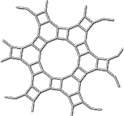DGCI'2006

## Discrete Geometry for Computer Imagery

### October 25-27, 2006 Szeged, Hungary## Invited Speakers

Jean-Marc Chassery, Grenoble

Duality and Geometry Straightness, Characterization and Envelope

Duality applied to geometrical problems is widely used in many applications in computer vision or computational geometry. A classical example is the Hough Transform to detect linear structures in images. In this lecture, we focus on two kinds of duality/polarity applied to geometrical problems: digital straightness detection and envelope computation.

T. Yung Kong, New York, IAPR Distinguished Speaker

Minimal Non-Simple and Minimal Non-Cosimple Sets in Binary Images on Cell

The concepts of weak component and simple 1 are generalizations, to binary images on the n-cells of n-dimensional cell complexes, of the standard concepts of "26-component" and "26-simple" 1 in binary images on the 3-cells of a 3D cubical complex; the concepts of strong component and cosimple 1 are generalizations of the concepts of "6-component" and "6-simple" 1. Over the past 20 years, the problems of determining just which sets of 1's can be minimal non-simple, just which sets can be minimal non-cosimple, and just which sets can be minimal non-simple (minimal non-cosimple) without being a weak (strong) foreground component have been solved for the 2D cubical and hexagonal, 3D cubical and face-centered-cubical, and 4D cubical complexes. This lecture solves these problems in much greater generality, for a very large class of cell complexes of dimension less than or equal to 4.

László Lovász, Budapest

Geometric representations and algorithms

The study of geometrically defined graphs, and of the reverse question, the construction of geometric representations of graphs, leads to unexpected connections between geometry, graph theory, and algorithms. A large variety of graph properties, seemingly with no relation to geometry, are related to the existence of certain geometric representations, and the construction of such representations leads, in turn, to efficient algorithms to test for these properties.